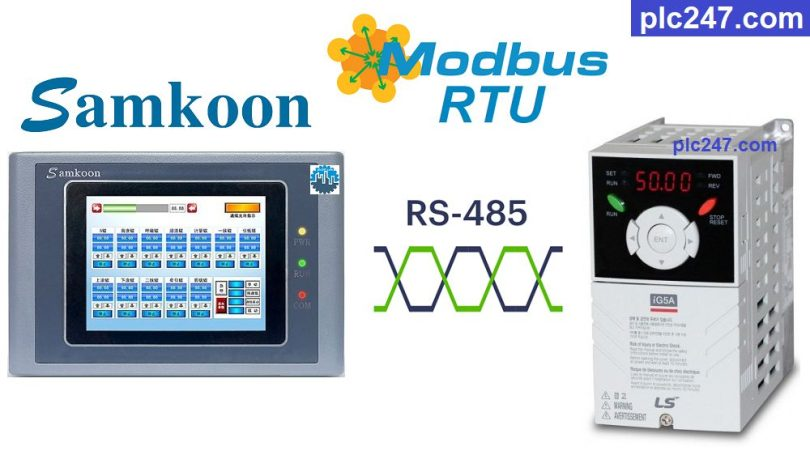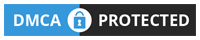# Samkoon HMI “Modbus RTU” LS iG5A VFD TutorialWritten by

Hello friends!
Continuing the series of articles on how to use Samkoon HMI to control different devices using the Modbus-RTU communication protocol, today plc247.com will guide you to control the LS iG5A inverter using this protocol.

Both the Samkoon HMI and the LS iG5A inverter have a built-in RS485 communication port for convenient Modbus-RTU connection.

#### Samkoon HMI & LS iG5A “Connection Diagram”

+ Below is the RS485 communication connection diagram between the Samkoon HMI and the LS iG5A Inverter

#### LS iG5A Modbus Parameters Setting

To be able to control the LS iG5A inverter using the Modbus-RTU protocol, we need to set the following communication parameters:

In this Project I install as follows:

+ Drv = 3
+ Frq = 7
+ I59 = 0 (Choose Modbus-RTU)
+ I60 = 1 (Slave No.1)
+ I61 = 3 (baudrate 9600bps)
+ I62 = 0
+ I65 = 2 (Even/1bit)

#### LS iG5A Modbus Registers Address

##### Note: LS iG5A Inverter Modbus RTU Address = “VFD Address – 1”

>>> Frequency Setting Address = 5 – 1 = 4 (dec)

>>> VFD Control Address = 6 – 1 = 5 (dec)

+ Set Word “5” = 2 >>> Run Forward
+ Set Word “5” = 4 >>> Run Reverse
+ Set Word “5” = 0 >>> Stop

>>> Current Output Address = 9 – 1 = 8 (dec)
>>> Frequency Output Address = A (hex) = 10 (dec) – 1 = 9 (dec)
>>> Voltage Output Address = B (hex) = 11 (dec) – 1 = 10 (dec)

#### HMI Samkoon “Modbus-RTU” Configuration

We need to set the communication parameters for the HMI exactly the same as the LS iG5A inverter

### HMI Programming

+ Interface Panel

+ Set Frequency Command

+ “Forward Run” Command

+ “Reverse Run” Command

+ Stop Command

### Project Video Tutorial

======

Related Software and Documents